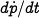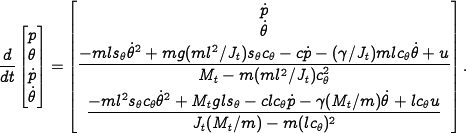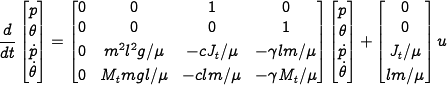# Errata: In Example 2.1, the state space equations for the balance system are missing a divisor of J t in one of the terms

In the state space equations for the balance systems example, the numerator of the equation foris missing a divisor ofin theterm. The correct equation isThis error propagates to the next equation, where theterm in the (3,4) entry of the dynamics matrix should be deleted. The correct expression is